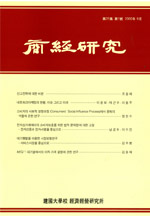상세검색
최근 검색어 전체 삭제
다국어입력
즐겨찾기0학술저널

# 로지스틱 회귀분석에서 편의추정방법에 대한 비교연구Alternative biased estimators in logistic regression

• 2000.03
• 153 - 164 (12 pages)

It is known that collinarity among the explanatory variables in logistic regression model inflates the variance of MLE. As an option to traditional MLE for logistic regression, biased estimators such as Ridge, Stein-type, Variable selection and NG are discussed. Furthermore, a new method, called combined type estimator, is proposed for istimation in logistic model. If the regression equations generated by a procedure do not change drastically with small change in the data, the procedure is called stable. Variable selection is instable, Ridge and Stein-type are very stable, and combined type estimator is intermediate. In case of MLE, Efron(1986) discussed the general measure of prediction crror which can be applied to GLM. In this paper, we adopt same idea to combines estimator and obtain a prediction-oriented criterion, which can be used in the choice of shrinkage matrix for logistic regression.

Ⅰ. 서론

Ⅱ. 혼합형태의 추정량(Combined type Estimator)

Ⅲ. 축소모수 결정을 위한 판단기준

Ⅳ. 결론

참고문헌

Abstract